Latest Banking jobs   »   IBPS quant

# Quantitative Aptitude Quiz For IBPS PO Prelims 2023 -25th August

Q1. A and B invested in a partnership Rs. 5000 and Rs. X for half and two-third of time respectively and profit share of A is 20% more than profit share of B. Find X?
(a) Rs. 3000
(b) Rs. 2000
(c) Rs. 2525
(d) Rs. 2715
(e) Rs. 3125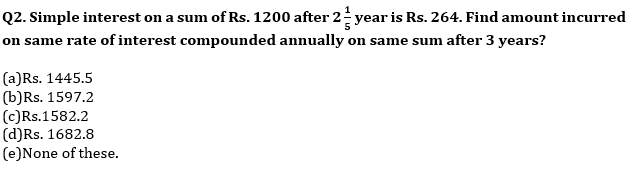Q3. Shivam invested some amount at SI and gets 45% more amount and the ratio between numerical value of rate of interest of Shivam’s investment to time period of Shivam’s investment is 5 : 1. If Bhuvan invested Rs. 6000 at the same rate and same period of time on CI, then find he will get how much amount?
(a) 9075.25 Rs
(b) 9175.25 Rs
(c) 8925.25 Rs
(d) 8975.25 Rs
(e) 9125.25 Rs

Q4. Bhavya prepare to lend 8000 Rs for 3 years at rate of 10% p.a. but at the time of lending he lends less amount due to which his total gain decreases by 450 Rs find the amount he reduced from the initial amount(in Rs)
(a) 6500
(b) 2000
(c) 1500
(d) 4000
(e) 2500

Q5. Find the sum if the difference between simple interest and compound interest on that sum of money for 3 years at 12.5% per annum is Rs 250.
(a) Rs 4210
(b) Rs 5120
(c) Rs 4260
(d) Rs 5210
(e) Rs 6230

Q6. A man invested Rs. X and Rs. 2X at 15% simple interest and at 8% compound interest (compounding annually) for two years respectively. Difference between simple interest and compound interest received after two years is Rs.820. Find the value of X.
(a) Rs.25000
(b) Rs.30600
(c) Rs.28600
(d) Rs.22200
(e) Rs.26200

Q7. A and B have equal amount, while A invested his amount on C.I. for two years at the rate of 10% p.a. and B invested 2/3 rd of his amount at the rate of R% p.a. on simple interest and remaining amount at the rate of 6.5% p.a. on also simple interest. If interest received by both at the end of two years are equal, then find the value of (R + 2.5) %?
(a) 12%
(b) 15%
(c) 17.5%
(d) 22.5%
(e) 12.5%

Q8. A mixture contains milk and water in the ratio 3:1. On adding 4 liters of water, the ratio of milk to water becomes 7:3. The quantity of water in the final mixture is?
(a) 16 liters
(b) 14 liters
(c) 18 liters
(d) 20 liters
(e) 24 liters

Q9. 50% of a mixture containing water and Sulphuric acid in ratio 1 : 2 is mixed with 40% of another mixture containing alcohol and water in ratio 3 : 2 so that ratio of alcohol: water : sulphuric acid becomes 12 : 13 : 10 in the final mixture. If sulphuric acid in initial mixture is 40 liter, Find total quantity of water and alcohol in final mixture?
(a) 25 liters
(b) 50 liters
(c) 75 liters
(d) 15 liters
(e) None of these

Q10. Ratio of two numbers is 5 : 7. If 30 is added in each number, the ratio becomes 3 : 4. Find the ratio of the number if 10 is subtracted from each number.
(a) 5 : 8
(b) 8 : 11
(c) 7 : 10
(d) 6 : 11
(e) 7 : 9

Solutions: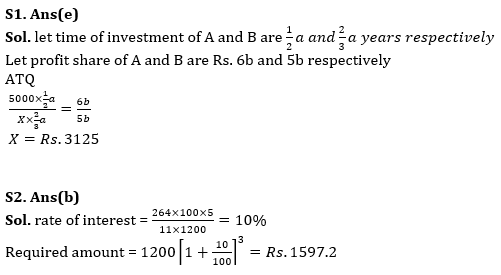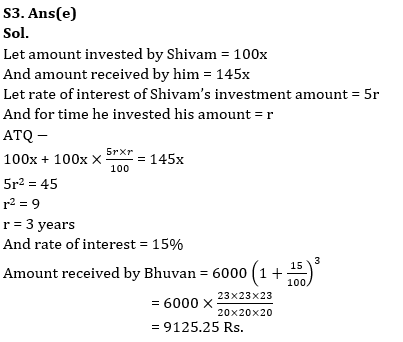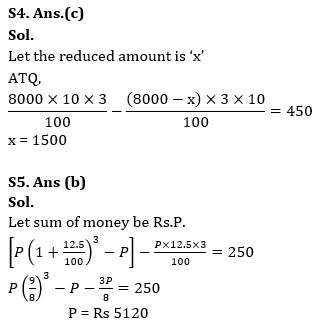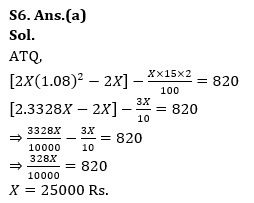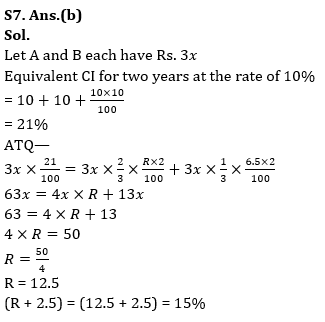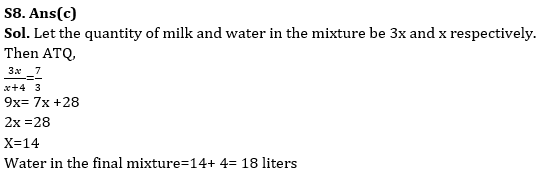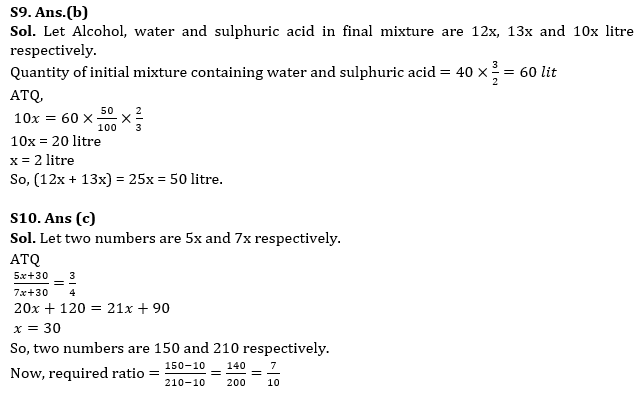## FAQs

### When will the IBPS PO prelims 2023 be conducted?

IBPS PO Prelims will be conducted on 23, 30 September, and 1 October 2023.

#### Congratulations!Union Budget 2023-24: Free PDF